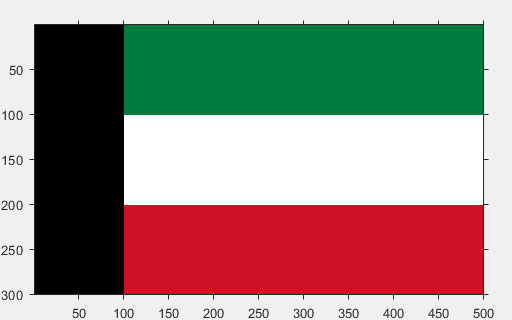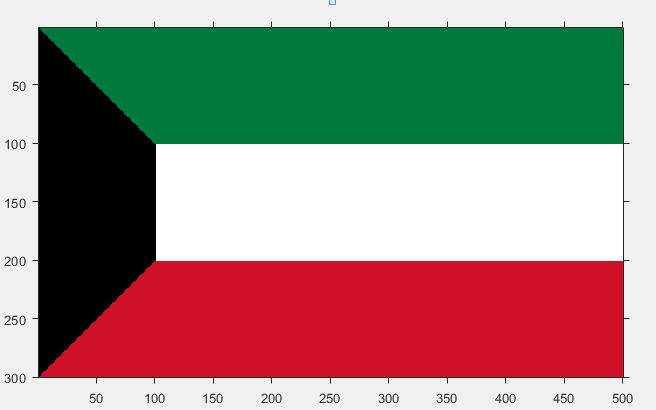# Draw Kuwait Flag using MATLAB

• Last Updated : 01 Mar, 2019

A colored image can be represented as a 3 order matrix. The first order is for the rows, the second order is for the columns and the third order is for specifying the color of the corresponding pixel. Here we use the RGB color format, so the third order will take 3 values of Red, Green ans Blue respectively. The values of the rows and columns depends on the size of the image.

Prerequisite : RGB image representation

Approach :

• Make a 3 order zero matrix of dimensions 300X500X3. 300 denotes the number of pixels for rows, 500 denotes number of pixels for columns and 3 denotes the color coding in RGB format. The image starts completely black because for all the pixels the color code is (0, 0, 0).
• Paint the horizontal bar between rows 1 to 100, and between columns 101 to 500 green. The color code of green in Kuwait’s flag is (0, 122, 61).
• Paint the horizontal bar between rows 101 to 200, and between columns 101 to 500 white. The color code of white is (255, 255, 255).
• Paint the horizontal bar between rows 201 to 300, and between columns 101 to 500 red. The color code of red in Kuwait’s flag is (206, 17, 38)
• At this point we have an image which looks like this:Now we have to draw 2 triangles.
• For the upper green triangle. In the matrix with rows from 1 to 100, and columns from 1 to 100, paint the upper right triangle green.
• For the lower red triangle. In the matrix with rows from 201 to 300, and columns from 1 to 100, paint the lower right triangle red.

Below is the implementation :

 `% MATLAB code to draw Kuwait flag`` ` `% initialising a zero matrix of 300X500X3``I=uint8(zeros(300, 500, 3));`` ` `% green horizontal bar``I(1:100, 101:500, 1)=0;``I(1:100, 101:500, 2)=122;``I(1:100, 101:500, 3)=61;`` ` `% white horizontal bar``I(101:200, 101:500, :)=255;``%red bar``I(201:300, 101:500, 1)=206;``I(201:300, 101:500, 2)=17;``I(201:300, 101:500, 3)=38;`` ` `% green upper triangle``for` `i = 1:100``    ``for` `j=1:100``        ``if` `i<=j``            ``I(i, j, 1)=0;``            ``I(i, j, 2)=122;``            ``I(i, j, 3)=61;``        ``end``    ``end``end`` ` `% red lower triangle``for` `i = 201:300``    ``for` `j = 1:100``        ``if` `(i-200)+j>=101``            ``I(i, j, 1)=206;``            ``I(i, j, 2)=17;``            ``I(i, j, 3)=38;``        ``end``    ``end``end`` ` `% displaying the matrix as image``figure, imshow(I);`

Output :My Personal Notes arrow_drop_up